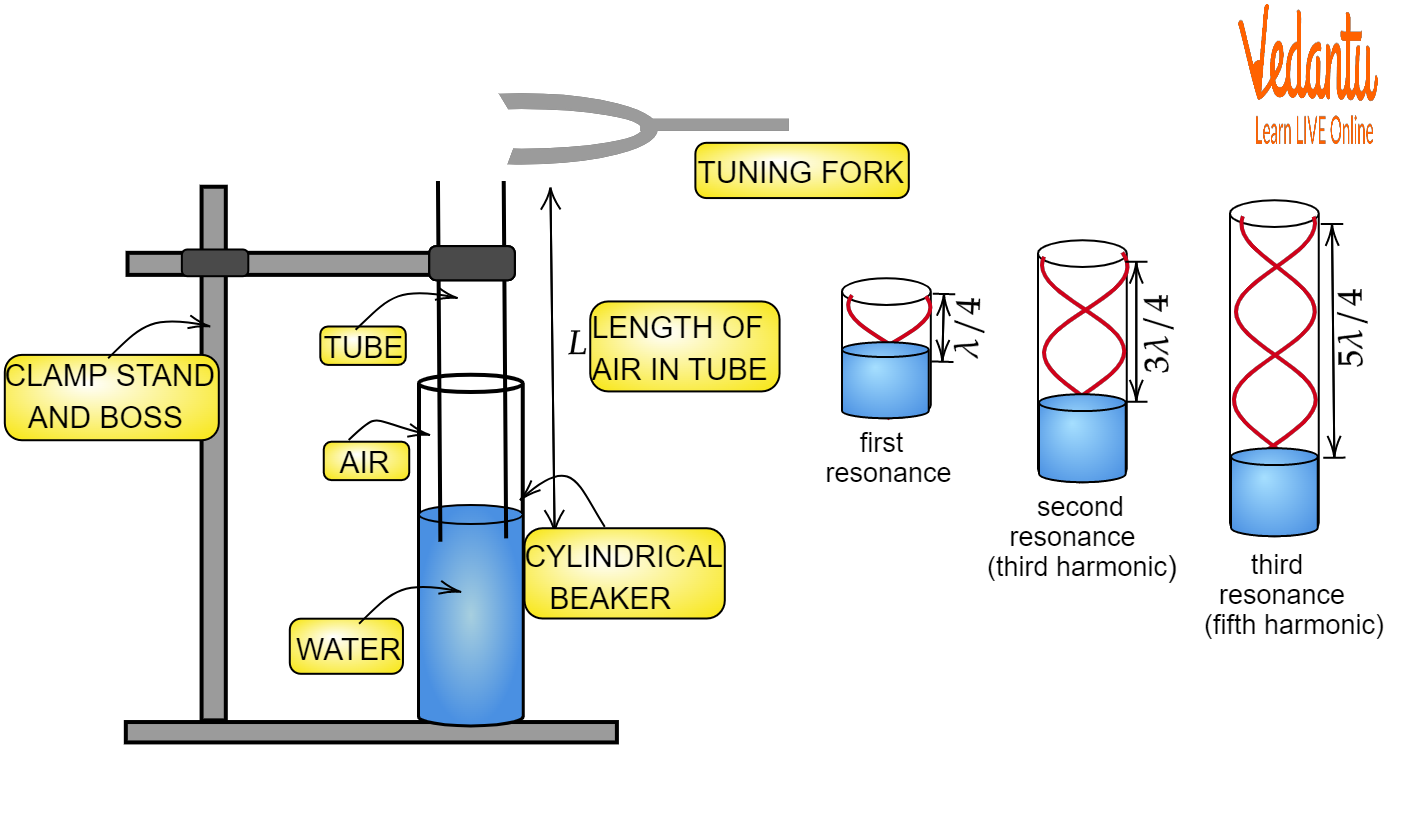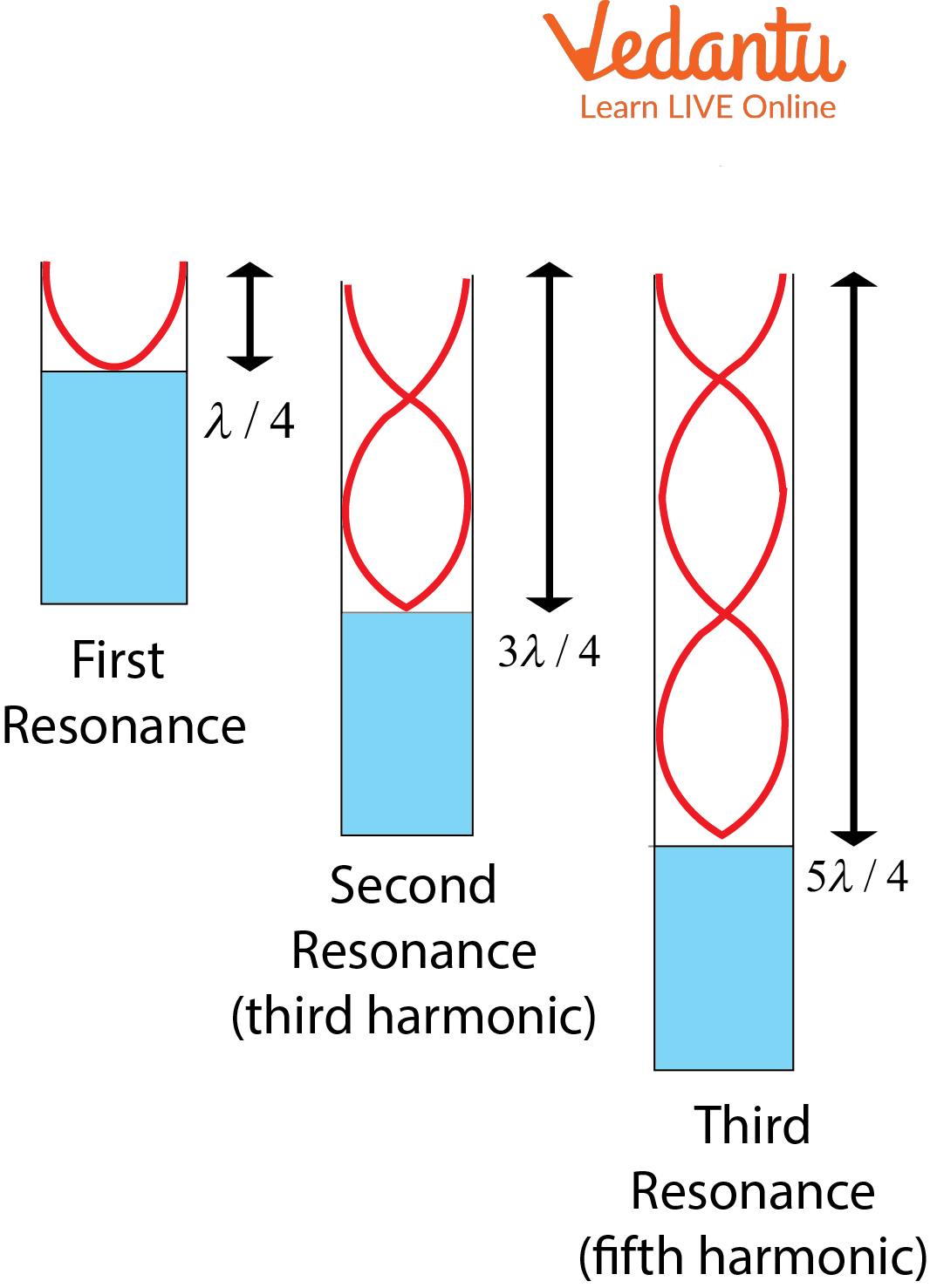Courses
Courses for Kids
Free study material
Free LIVE classes
More

# Resonance Column Tube - JEE Important TopicLIVE
Join Vedantu’s FREE Mastercalss

## What is a Resonance Column?

The resonance of an air column helps to determine the wavelength of the sound. The apparatus used for the experiment consists of a long cylindrical tube that is attached to the water reservoir. The resonance is indicated by the increase in the intensity of the sound.

The stationary wave is produced by the superposition of two waves of the same frequency and amplitude which are traveling with the same velocity in opposite directions. These waves produce certain fixed points along with the medium which undergoes zero displacements. These points where there is no displacement are called nodes. Somewhere, in the middle between all nodes are the regions of maximum displacement. Thus, these points are called antinodes.

## Resonance Column Apparatus

The resonance air column apparatus is one of the simplest techniques used to measure the speed of sound in air at room temperature. The vibration observed in the experiment can be set up in a resonance column apparatus. This resonance is a standing wave phenomenon in the air column. The resonance column apparatus consists of a long metal tube which is held vertically in a tall jar which contains water. This tube can also be fixed in a vertical position, also the length of the column can be varied by raising or lowering the tube.

• Resonance tube

• Thermometer

• Set Squares

• Plumb Line

• Water in a beaker

• Two-timing fork of known frequency

• Plastic beakersResonance Tube Apparatus

## Resonance Experiment Tube

The tube used in the experiment is filled with water. In the due course of the experiment, resonance is observed in the tube which is indicated by the sudden increase in the intensity of the sound. This resonance helps to determine the wavelength. The water level is moved up and down several times to locate the point of maximum sound intensity. The resonance counted in a certain distance of the tube is thus used to determine the wavelength and velocity of the sound. Now, let us understand the experiment a bit more clearly.

When the vibrating tuning fork is held above the open end, we can observe that longitudinal waves are sent down the air column. These waves are then reflected towards the water surface and thus produce standing waves. Here, the surface of water acts as the closed end. Also, nodes are produced at the water surface and antinodes are produced at the open end.Waveform Construction in the Resonance Tube Experiment

Now, when the natural frequency of the tuning fork is equal to the frequency of the waves in the air column, a loud sound is produced in the column. This is the condition of resonance. This condition only occurs when the length of the air column is proportional to the one-fourth of the wavelength of the sound waves, where the frequency is equal to the frequency of tuning fork.

For first resonance,

${{l}_{1}}=\frac{\lambda }{4}$

Which means, for second resonance,

${{l}_{1}}=\frac{3\lambda }{4}$

From the above both equations, we get the relation

$\lambda =2\left( {{l}_{2}}-{{l}_{1}} \right)$

Therefore, at room temperature, the velocity of sound in air column can be found as

\begin{align} & u=v\lambda \\ & u=2v\left( {{l}_{2}}-{{l}_{1}} \right) \\ \end{align}

The velocity of sound at ${{0}^{\circ }}C$ can be calculated as

$v=v\sqrt{\frac{273}{273+t}}$

## End Correction of a Resonance Column

The short distance which is applied or added to the real length of a resonance tube, which helps to calculate the precise resonance frequency of the pipe is known as the end correction of the resonance column. A precise value of the resonance frequency is estimated when end correction is applied. It is to note that the fundamental resonance of a pipe occurs when the length of the resonator is half of the sound wavelength or quarter of the sound wavelength. The practical frequency of resonance comes out lower than this, thus we need to apply an end correction, thus the pipe used in the experiment is somewhat longer than its physical length. A precise value of resonance frequency of the pipe used in the experiment can be estimated, when an end correction is applied.

## Conclusion

The wavelength of sound and the velocity of sound can be easily determined by the resonance tube experiment. This is based on the concept of stationary waves which get produced when two waves traveling at the same amplitude and same frequency in the opposite direction superimpose on each other. Resonance, which is a phenomenon of standing waves or stationary waves, can be used to find the velocity of sound. In order to attain an accurate value it is advisable to apply end correction to the original results.

Last updated date: 23rd Sep 2023
Total views: 116.1k
Views today: 2.16k

## FAQs on Resonance Column Tube - JEE Important Topic

1. Is the resonance column open pipe or closed pipe?

In the resonance column apparatus, a resonance tube is used whose one end is open and the end is closed. This apparatus is used to determine the speed and wavelength of the sound waves. The tube which is present in the apparatus is filled with water and thus the water surface acts as a closed end. A tuning fork which is present on the open end of the tube and is then vibrated which then produces standing waves in the air column. Therefore, the resonance column is both an open and closed system.

2. Why is water used in resonance tubes?

The water fills the space that is not filled by the air. It gives a way to control the exact length of the air column, so when the natural frequency of tuning fork is equal to the frequency of the waves in the air column, the loud sound made in the air column can be heard for maximum amplification, which therefore, means a resonance point have been found at one of the key points for measuring the sound wavelength.